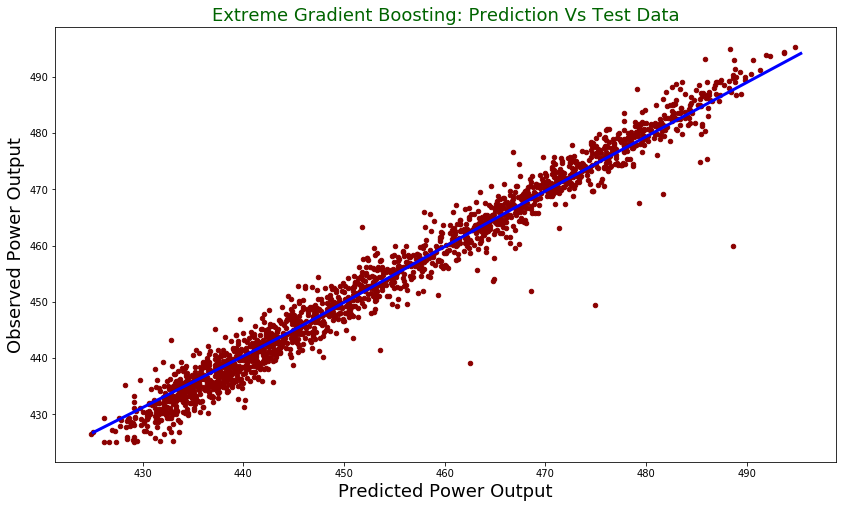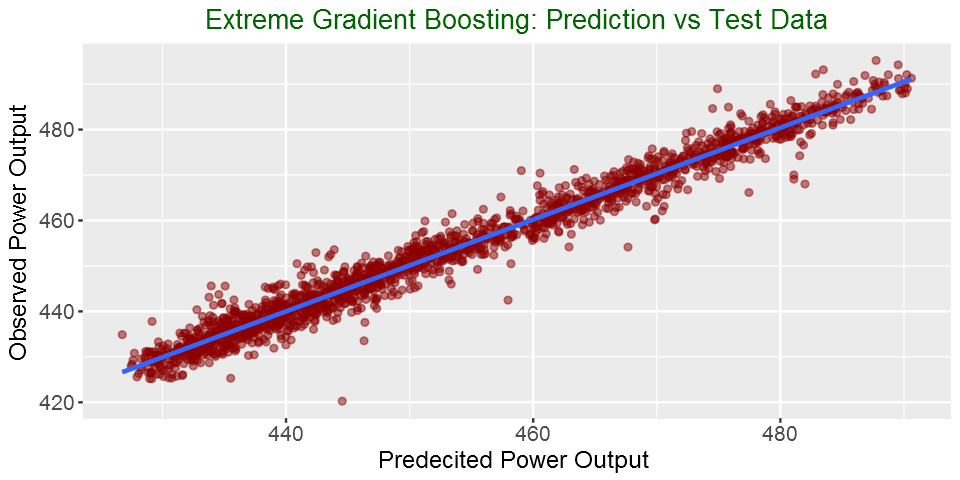Fisseha Berhane, PhD

Extreme Gradient Boosting (XGBoost) with R and Python¶

Extreme Gradient Boosting is among the hottest libraries in supervised machine learning these days. It supports various objective functions, including regression, classification and ranking. It has gained much popularity and attention recently as it was the algorithm of choice for many winning teams of a number of machine learning competitions. What makes it so popular are its speed and performance. It gives among the best performances in many machine learning applications. It is optimized gradient-boosting machine learning library. The core algorithm is parallelizable and hence it can use all the processing power of your machine and the machines in your cluster. In R, according to the package documentation, since the package can automatically do parallel computation on a single machine, it could be more than 10 times faster than existing gradient boosting packages.

xgboost shines when we have lots of training data where the features are numeric or mixture of numeric and categorical fields. It is also important to note that xgboost is not the best algorithm out there when all the features are categorical or when the number of rows is less than the number of fields (columns).

For other applications such as image recognition, computer vision or natural language processing, xgboost is not the ideal library. Do not use xgboost for small size dataset. It has libraries in Python, R, Julia, etc. In this post, we will see how to use it in R and Python.

This post is a continuation of my previous Machine learning with Python and R blog post series. The first one is available here. Data exploration was performed in the first part, so I will not repeat it here.

With Python¶

Import Python libraries¶

In :
import xgboost as xgb
import pandas as pd
import numpy as np
import statsmodels.api as sm
from sklearn.model_selection import train_test_split
from sklearn.model_selection import GridSearchCV
from sklearn.metrics import mean_squared_error
import matplotlib.pyplot as plt

Read the data into a Pandas dataframe

In :

Create training and test datasets

In :
X = power_plant.drop("PE", axis = 1)
y = power_plant['PE'].values
y = y.reshape(-1, 1)

X_train, X_test, y_train, y_test = train_test_split(X, y,test_size = 0.2, random_state=42)

Convert the training and testing sets into DMatrixes: DMatrix is the recommended class in xgboost.

In :
DM_train = xgb.DMatrix(data = X_train,
label = y_train)

DM_test =  xgb.DMatrix(data = X_test,
label = y_test)

There are different hyperparameters that we can tune and the parametres are different from baselearner to baselearner. In tree based learners, which are the most common ones in xgboost applications, the following are the most commonly tuned hyperparameters:

learning rate: learning rate/eta- governs how quickly the model fits the residual error using additional base learners. If it is a smaller learning rate, it will need more boosting rounds, hence more time, to achieve the same reduction in residual error as one with larger learning rate. Typically, it lies between 0.01 - 0.3

The three hyperparameters below are regularization hyperparameters.

gamma: min loss reduction to create new tree split. default = 0 means no regularization.

lambda: L2 reg on leaf weights. Equivalent to Ridge regression.

alpha: L1 reg on leaf weights. Equivalent to Lasso regression.

max_depth: max depth per tree. This controls how deep our tree can grow. The Larger the depth, more complex the model will be and higher chances of overfitting. Larger data sets require deep trees to learn the rules from data. Default = 6.

subsample: % samples used per tree. This is the fraction of the total training set that can be used in any boosting round. Low value may lead to underfitting issues. A very high value can cause over-fitting problems.

colsample_bytree: % features used per tree. This is the fraction of the number of columns that we can use in any boosting round. A smaller value is an additional regularization and a larger value may be cause overfitting issues.

n_estimators: number of estimators (base learners). This is the number of boosting rounds.

In both R and Python, the default base learners are trees (gbtree) but we can also specify gblinear for linear models and dart for both classification and regression problems.

In this post, I will optimize only three of the parameters shown above and you can try optimizing the other parameters. You can see the list of parameters and their details from the website.

Parameters for grid search

In :
gbm_param_grid = {
'colsample_bytree': np.linspace(0.5, 0.9, 5),
'n_estimators':[100, 200],
'max_depth': [10, 15, 20, 25]
}

Instantiate the regressor

In :
gbm = xgb.XGBRegressor()

Perform grid search

Let's perform 5 fold cross-validation using mean square error as a scoring method.

In :
grid_mse = GridSearchCV(estimator = gbm, param_grid = gbm_param_grid, scoring = 'neg_mean_squared_error', cv = 5, verbose = 1)

Fit grid_mse to the data, get best parameters and best score (lowest RMSE)

In :
grid_mse.fit(X_train, y_train)

print("Best parameters found: ",grid_mse.best_params_)
print("Lowest RMSE found: ", np.sqrt(np.abs(grid_mse.best_score_)))
Fitting 5 folds for each of 40 candidates, totalling 200 fits
[Parallel(n_jobs=1)]: Done 200 out of 200 | elapsed: 11.0min finished
Best parameters found:  {'colsample_bytree': 0.80000000000000004, 'max_depth': 15, 'n_estimators': 200}
Lowest RMSE found:  3.03977094354

Predict using the test data

In :
pred = grid_mse.predict(X_test)

print("Root mean square error for test dataset: {}".format(np.round(np.sqrt(mean_squared_error(y_test, pred)), 2)))
Root mean square error for test dataset: 2.76
In :
test = pd.DataFrame({"prediction": pred, "observed": y_test.flatten()})

lowess = sm.nonparametric.lowess

z = lowess(pred.flatten(), y_test.flatten())

test.plot(figsize = [14,8],
x ="prediction", y = "observed", kind = "scatter", color = 'darkred')

plt.title("Extreme Gradient Boosting: Prediction Vs Test Data", fontsize = 18, color = "darkgreen")

plt.xlabel("Predicted Power Output", fontsize = 18)

plt.ylabel("Observed Power Output", fontsize = 18)

plt.plot(z[:,0], z[:,1], color = "blue", lw= 3)

plt.show()With R¶

Now, let's build xgboost model with R. We will use the caret package for cross-validation and grid search.

In :
library(tidyverse)
library(xgboost)
library(caret)

In :

create training set indices with 80% of data: we are using the caret package to do this

In :
set.seed(100)  # For reproducibility

# Create index for testing and training data
inTrain <- createDataPartition(y = power_plant$PE, p = 0.8, list = FALSE) # subset power_plant data to training training <- power_plant[inTrain,] # subset the rest to test testing <- power_plant[-inTrain,] Convert the training and testing sets into DMatrixes: DMatrix is the recommended class in xgboost. In : X_train = xgb.DMatrix(as.matrix(training %>% select(-PE))) y_train = training$PE

X_test = xgb.DMatrix(as.matrix(testing %>% select(-PE)))
y_test = testing$PE Specify cross-validation method and number of folds. Also enable parallel computation In : xgb_trcontrol = trainControl( method = "cv", number = 5, allowParallel = TRUE, verboseIter = FALSE, returnData = FALSE ) This is the grid space to search for the best hyperparameters I am specifing the same parameters with the same values as I did for Python above. The hyperparameters to optimize are found in the website. In : xgbGrid <- expand.grid(nrounds = c(100,200), # this is n_estimators in the python code above max_depth = c(10, 15, 20, 25), colsample_bytree = seq(0.5, 0.9, length.out = 5), ## The values below are default values in the sklearn-api. eta = 0.1, gamma=0, min_child_weight = 1, subsample = 1 ) Finally, train your model In : set.seed(0) xgb_model = train( X_train, y_train, trControl = xgb_trcontrol, tuneGrid = xgbGrid, method = "xgbTree" ) Best values for hyperparameters In : xgb_train$bestTune
nroundsmax_depthetagammacolsample_bytreemin_child_weightsubsample
1820015 0.10 0.81 1

We see above that we get the same hyperparameter values from both R and Python.

Model evaluation

In :
predicted = predict(xgb_model, X_test)
residuals = y_test - predicted
RMSE = sqrt(mean(residuals^2))

cat('The root mean square error of the test data is ', round(RMSE,3),'\n')
The root mean square error of the test data is  2.856
In :
y_test_mean = mean(y_test)

# Calculate total sum of squares
tss =  sum((y_test - y_test_mean)^2 )

# Calculate residual sum of squares

# Calculate R-squared
cat('The R-square of the test data is ', round(rsq,3), '\n')
The R-square of the test data is  0.972

Plotting actual vs predicted

In :
options(repr.plot.width=8, repr.plot.height=4)
In :
my_data = as.data.frame(cbind(predicted = predicted,
observed = y_test))

# Plot predictions vs test data

ggplot(my_data,aes(predicted, observed)) + geom_point(color = "darkred", alpha = 0.5) +
geom_smooth(method=lm)+ ggtitle('Linear Regression ') + ggtitle("Extreme Gradient Boosting: Prediction vs Test Data") +
xlab("Predecited Power Output ") + ylab("Observed Power Output") +
theme(plot.title = element_text(color="darkgreen",size=16,hjust = 0.5),
axis.text.y = element_text(size=12), axis.text.x = element_text(size=12,hjust=.5),
axis.title.x = element_text(size=14), axis.title.y = element_text(size=14))Summary¶

In this post, We used Extreme Gradient Boosting to predict power output. We see that it has better performance than linear model we tried in the first part of the blog post series. The RMSE with the test data decreased from more 4.4 to 2.8. See you in the next part of my machine learning blog post.